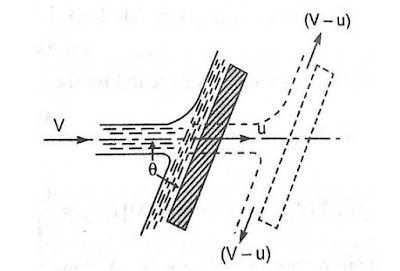# The inclined plate moving of jet - Ishwaranand

Follow Your Dream | Believe in YourSelf | & Don't Give Up

## Force on the inclined plate moving in the direction of the jetImage Source ~ Crafted With ©Ishwaranand - 2020 ~ Image by ©Ishwaranand
Fig. Jet striking an inclined moving plate
Let
v = absolute velocity of a jet of water,
u = velocity from the plate into the direction about the jet,
a = Cross-sectional area of jet, and
θ = Angle between jet and plate.
If the plate is smooth and loss of energy due to impact of the jet is assumed zero, the jet of water will leave the inclined plate with a velocity equal to (v- u).

The force exerted by the jet of water on the plate in the direction normal to the plate is given as
Fn = Mass striking per second x [Initial velocity in the normal direction with which jet strikes - Final velocity]
= ρa (v - u) [(v - u) sinθ - 0]
= ρa (v - u)^2 sinθ
• This normal force Fn is resolved into two components namely Fx and Fy in the direction of the jet and perpendicular to the direction of the jet respectively.
Fx = Fn sinθ
= ρa (v - u)^2 sin^2θ
Fy = Fn cosθ
= ρa (v - u)^2 sinθ cosθ

= Fx x Distance per second in the direction of x
= Fx x u
= ρa(v - u)^2 sin^2θ x u
= ρa (v - u)^2 u sin^2θ N m/s.

Ex. A jet of water of diameter 10 cm strikes a flat plate normally with a velocity of 15 m/s. Each plate is moving by a velocity of 6 m/s in some direction of the jet also away from the jet.
Find
1. This forces exerted by the jet at the plate
2. Work is done by the jet on the plate per second
3. Power of jet in kW
4. Efficiency of jet
Given
The diameter of the jet,
d = 10 cm = 0.1 m,
Area,
a = (π/4)d^2
= (π/4)(0.1)^2
= 0.007854 m^2
The velocity of jet,
v = 15 m/s
The velocity of the plate,
u = 6 m/s.
Solution
This force exerted by the jet to a moving flat vertical plate is given by,
Fx = ρa (v - u)^2
= 1000 x 0.007854 x (15 - 6)^2
= 636.17 N
Work done per second by the jet
= Fx x u
= 636.17 x 6
= 3817.02 Nm/s
Power of jet
= (work is done per second)/1000
= 3817.02/1000
= 3.817 kW
Efficiency of jet (η)
= Output of jet per second/Input of jet per second
Where,
output of jet per second  = work done by jet per second
= 3817.02 Nm/s and
input of jet per second = Kinetic energy of the jet per second
= (1/2) m v2 /s
= (1/2) (m/s) v2
= (1/2) (ρav) v2
= (1/2) ρav^3
= (1/2) 1000 x 0.007854 x 15^3
= 13253.6 Nm/s
= 3817.02/13253.6
= 0.288
= 28.8 %